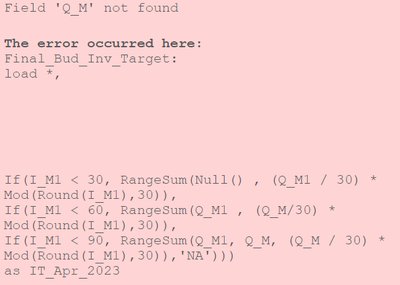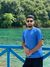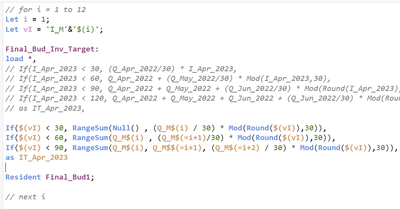Announcements
Applications are open for the 2024 Qlik Luminary Program. Apply by December 15 here.
cancel
Showing results for
Did you mean:Creator

## Variable expansion is not working in Rangesum()

Hi all, I am new to the qlik sense. I have created a variable i = 1 and used in the calculated dimension as below.

Let i = 1;
Let vI = 'I_M'&'\$(i)'; // I_M1

If(\$(vI) < 30, RangeSum(       (Q_M\$(i) / 30) * Mod(Round(\$(vI)),30),
If(\$(vI) < 60, RangeSum(        Q_M\$(i) ,        (Q_M\$(=i+1)/30) * Mod(Round(\$(vI)),30)),
If(\$(vI) < 90, RangeSum(        Q_M\$(i),         Q_M\$(=i+1),           (Q_M\$(=i+2) / 30) * Mod(Round(\$(vI)),30)),'NA')))
as IT_Apr_2023

The above expansion should look like this;

If(I_M1 < 30, RangeSum(       (Q_M1 / 30) * Mod(Round(I_M1),30),
If(I_M1 < 60, RangeSum(        Q_M1 ,        (Q_M2 / 30) * Mod(Round(I_M1),30)),
If(I_M1 < 90, RangeSum(        Q_M1,         Q_M2,           (Q_M3 / 30) * Mod(Round(I_M1),30)),       'NA')))
as IT_Apr_2023

But it is giving error as below.Q_M\$(i) is expanding like Q_M1. But Q_M\$(=i+1) is throwing error like 'Q_M' field is not found.

Labels (4)

• ### Variables

1 Solution

Accepted SolutionsMVP & Luminary

Within the script is only the single \$-expansion, like: \$(var) available but not the double one, like: \$(=var).

In some scenarios - in which the call expects respectively accepts a calculation - you may use something like:

\$(var) + Number

respectively with a little force:

(\$(var) + Number)

You may try it but I think it won't work within your scenario because Qlik expects to get valid field-names within the load which a Field\$(var) would be because the call of \$(var) means just a replacement with the assigned variable-value but not a Field & EvaluationOfWhatever because it will always results in a string-value and not accepted as field-name even if it look like that.

Therefore I think what you are trying isn't possible - at least not in this way. If you want to remain quite closely to this approach the simplest way seems to be to use more variables, like i and ii which is just i + 1. Beside this I'm not sure if your shown loop from 1 to 12 will be really satisfying because this logic won't be able to handle a year-switch.

In general I suggest to re-thing the entire approach because your source is a crosstable and this data-structure has a lot of disadvantages especially by the handling of the multiple fields and even more if they are unknown and/or have a unknown amount of fields and/or any dynamic accessing is needed. This means it's mostly much better to transform the crosstable into a "normal" data-structure and applying everything needed calculations on them (in your case maybe with an ordered resident-load and using interrecord-functions like peek() and previous() to interact with surrounding rows).

15 RepliesPartner - Contributor III

Hi @Lokesh_5045

In Qlik Sense, variable expansion using \$(=) syntax is not supported inside functions like RangeSum(). The variable expansion happens during the script execution phase, and it is not evaluated dynamically within the expression.

To achieve the desired result, you can use an alternative approach by creating a series of IF statements to handle the different conditions based on the value of 'i'.

Here's an example of how you can modify your expression to achieve the desired result:

```If(\$(vI) < 30, RangeSum( (Q_M1 / 30) * Mod(Round(\$(vI)),30), If(\$(vI) < 60, RangeSum( Q_M1 , (Q_M2 / 30) * Mod(Round(\$(vI)),30)), If(\$(vI) < 90, RangeSum( Q_M1, Q_M2, (Q_M3 / 30) * Mod(Round(\$(vI)),30)), 'NA'))) as IT_Apr_2023```

In this modified expression, you explicitly specify the field names (Q_M1, Q_M2, Q_M3) instead of using the variable expansion. This approach ensures that the correct field names are used in the expression, regardless of the value of 'i'.

Please note that if you have a large number of conditions or need to handle more dynamic scenarios, you might consider using a scripting approach to dynamically generate the expression based on the value of 'i'.

Regards, Mohamed Ibrahim.Creator
Author

But I am using this in a for loop. For every loop I am creating new field. I forgot to rename the field at the end. It will be renamed by IT_M1.

There will be more fields IT_M2, IT_M3..... up to 12. In each loop 'i' value is changing.

In first loop, Rangesum(Q1, Q2, Q3) when i = 1.
In second loop, it becomes Rangesum(Q2, Q3, Q4) when i = 2
My question was when Q_M\$(i) is expanding as Q_M1 and Q_M\$(=i+1) is not expanding as Q_M2. How can I achieve this?

Let me show you the complete code.Hope you understood why I am using 'i'. I can't write the same code 12 times. I need to use for loop.Partner - Contributor III

@Lokesh_5045  Try this

If('I_M'&\$(i) < 30, RangeSum((Q_M\$(i) / 30) * Mod(Round('I_M'&\$(i)), 30),
If('I_M'&\$(i) < 60, RangeSum(Q_M\$(i), (Q_M\$(i+1) / 30) * Mod(Round('I_M'&\$(i)), 30)),
If('I_M'&\$(i) < 90, RangeSum(Q_M\$(i), Q_M\$(i+1), (Q_M\$(i+2) / 30) * Mod(Round('I_M'&\$(i)), 30)),
'NA')))
as IT_Apr_2023

Regards, Mohamed Ibrahim.Creator
Author

@snibrahim1993 I have tried that one before. But to make sure, I tried it again just now. Same error is popping up as mentioned in the problem statement.Partner - Contributor III

@Lokesh_5045 Have you tried Field value funcstion?
If(FieldValue('I_M' & \$(i), 1) < 30,RangeSum((Q_M\$(i) / 30) * Mod(Round(FieldValue('I_M' & \$(i), 1)), 30),
If(FieldValue('I_M' & \$(i), 1) < 60,RangeSum(Q_M\$(i), (Q_M\$(= \$(i)+1) / 30) * Mod(Round(FieldValue('I_M' & \$(i), 1)), 30)),
If(FieldValue('I_M' & \$(i), 1) < 90,RangeSum(Q_M\$(i), Q_M\$(= \$(i)+1), (Q_M\$(= \$(i)+2) / 30) * Mod(Round(FieldValue('I_M' & \$(i), 1)), 30)),
'NA')))
as IT_Apr_2023

Regards, Mohamed Ibrahim.Creator
Author

@snibrahim1993 Sorry for late reply. It didn't work. Same error is appearing like 'Q_M' field is not found.Partner - Contributor III

Let i = 1;
Let vI = 'I_M' & \$(i);

If(\$(vI) < 30,RangeSum((Q_M\$(i) / 30) * Mod(Round(\$(vI)),30),
If(\$(vI) < 60,RangeSum(Q_M\$(i),\$(= 'Q_M' & Num(i+1)) / 30 * Mod(Round(\$(vI)),30)),
If(\$(vI) < 90,RangeSum(Q_M\$(i),Q_M\$(=i+1),\$(= 'Q_M' & Num(i+2)) / 30 * Mod(Round(\$(vI)),30)
),
'NA'
)
)
),
'NA'
) as IT_Apr_2023

Regards, Mohamed Ibrahim.Partner - Contributor III

@Lokesh_5045  Try the above script otherwise please share the exact logic code and expected result

Regards, Mohamed Ibrahim.MVP & Luminary

Within the script is only the single \$-expansion, like: \$(var) available but not the double one, like: \$(=var).

In some scenarios - in which the call expects respectively accepts a calculation - you may use something like:

\$(var) + Number

respectively with a little force:

(\$(var) + Number)

You may try it but I think it won't work within your scenario because Qlik expects to get valid field-names within the load which a Field\$(var) would be because the call of \$(var) means just a replacement with the assigned variable-value but not a Field & EvaluationOfWhatever because it will always results in a string-value and not accepted as field-name even if it look like that.

Therefore I think what you are trying isn't possible - at least not in this way. If you want to remain quite closely to this approach the simplest way seems to be to use more variables, like i and ii which is just i + 1. Beside this I'm not sure if your shown loop from 1 to 12 will be really satisfying because this logic won't be able to handle a year-switch.

In general I suggest to re-thing the entire approach because your source is a crosstable and this data-structure has a lot of disadvantages especially by the handling of the multiple fields and even more if they are unknown and/or have a unknown amount of fields and/or any dynamic accessing is needed. This means it's mostly much better to transform the crosstable into a "normal" data-structure and applying everything needed calculations on them (in your case maybe with an ordered resident-load and using interrecord-functions like peek() and previous() to interact with surrounding rows).Tags
Community Browser